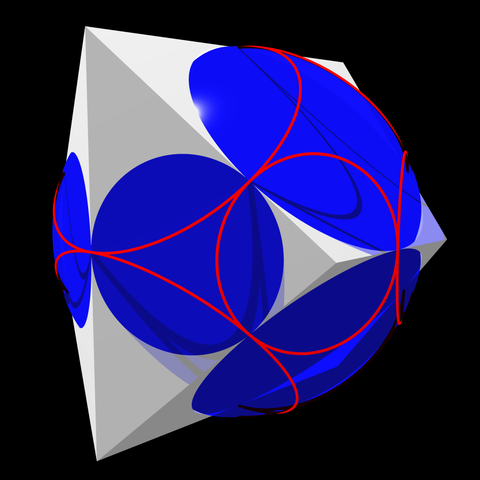One of the desirable properties of graph drawing algorithms is “symmetry display”: the drawing should reflect some of the symmetries of the input graph. This can be achieved either by determining the symmetries and then placing vertices according to those symmetries, or in some cases by algorithms that automatically produce a symmetric output. For instance, the canonical polyhedron (a polyhedron with all edges tangent to the unit sphere and with the centroid of these tangencies at the center of the sphere) automatically realizes all symmetries of any given polyhedral graph. The image below shows an example. In the image, the red rings are the horizons of visibility on the blue sphere from each peak. The blue disks poking through the faces of the polyhedron, and the red rings, give two orthogonal circle packings representing the graph of the polyhedron and its dual.What symmetry groups are possible? All of them! Frucht’s theorem, published by Robert Frucht in 1939, states that every finite group is the group of symmetries of a finite undirected graph. Ten years later, Frucht proved more strongly that the graph realizing any symmetry group can be chosen to be 3-regular.

But if we restrict our attention to other special classes of graphs, the answer is very different. Trees, for instance, do not ever have the cyclic group of order three as their group of symmetries. Babai proved in 1972 that planar graphs also cannot realize all symmetry groups; later, he characterized the symmetry groups of planar graphs and proved more generally that every minor-closed graph family has a symmetry it cannot realize. It’s not a matter of having only small groups of symmetries; for instance the graphs $$K_{1,n}$$ are trees (and planar graphs) with the large symmetric group $$S_n$$ as their symmetries. And every finite group can be represented as a permutation group, a subgroup of the symmetric group. But trimming off the other symmetries to leave only the desired finite group and not the rest of the symmetric group can be difficult.

If planar graphs don’t have all possible symmetries, what about 1-planar graphs, the graphs that can be drawn with one crossing per edge? In this case, as in Frucht’s theorem, all symmetries are indeed possible. To see this, use Frucht’s theorem to realize your favorite finite group as the symmetries of a finite graph $$G$$ (making sure that $$G$$ has no degree-two vertices, possible by the 3-regular version of Frucht’s theorem). Draw $$G$$ in the plane, with some maximum number $$k$$ of crossings per edge. Then the graph $$G'$$ formed by replacing each edge of $$G$$ by a $$k$$-edge path is 1-planar, and has the same symmetries as $$G$$.

The drawing below shows an example of this process. I started with a graph whose symmetry group is the generalized quaternion group $$Q_{16}$$, one of the ones that Babai’s original 1972 result showed must be nonplanar. I found this graph in Graves et al. “Smallest graphs with given generalized quaternion automorphism group”; in the drawing below, its vertices are the blue ones of degree five and the yellow ones of degree three. I redrew the same graph with two crossings per edge (the drawing by Graves et al. had many more), and then added a subdivision point on each edge (the red vertices) to make it 1-planar, while keeping the same $$Q_{16}$$ symmetry group. Unfortunately the drawing doesn’t show off much of the symmetry of the graph, but that’s inevitable, because its symmetry group is not one of the ones that can be realized by a planar drawing.Since 1-planar graphs have polynomial expansion, this shows that the graphs of polynomial expansion are like the nowhere-dense graphs (which include the 3-regular graphs), and unlike the minor-closed graph families, in allowing every symmetry group to be realized.